# JP2006279917A - Dynamic image encoding device, dynamic image decoding device and dynamic image transmitting system - Google Patents

Dynamic image encoding device, dynamic image decoding device and dynamic image transmitting system Download PDF

## Info

Publication number
JP2006279917A
JP2006279917A JP2005306267A JP2005306267A JP2006279917A JP 2006279917 A JP2006279917 A JP 2006279917A JP 2005306267 A JP2005306267 A JP 2005306267A JP 2005306267 A JP2005306267 A JP 2005306267A JP 2006279917 A JP2006279917 A JP 2006279917A
Authority
JP
Japan
Prior art keywords
image
frame
motion vector
moving image
interpolation
Prior art date
Legal status (The legal status is an assumption and is not a legal conclusion. Google has not performed a legal analysis and makes no representation as to the accuracy of the status listed.)
Pending
Application number
JP2005306267A
Other languages
Japanese (ja)
Inventor
Kotaro Asai
Yuichi Izuhara
Shunichi Sekiguchi
Kazuo Sugimoto

Original Assignee
Mitsubishi Electric Corp

Priority date (The priority date is an assumption and is not a legal conclusion. Google has not performed a legal analysis and makes no representation as to the accuracy of the date listed.)
Filing date
Publication date
Priority to JP2005061056 priority Critical
Application filed by Mitsubishi Electric Corp, 三菱電機株式会社 filed Critical Mitsubishi Electric Corp
Priority to JP2005306267A priority patent/JP2006279917A/en
Publication of JP2006279917A publication Critical patent/JP2006279917A/en
Application status is Pending legal-status Critical

• 230000015654 memory Effects 0 abstract 4
• 238000000034 methods Methods 0 abstract 2
• 241001442055 Vipera berus Species 0 abstract 1
• 238000006243 chemical reaction Methods 0 abstract 1
• 230000002829 reduced Effects 0 abstract 1

## Images

•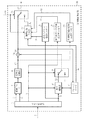•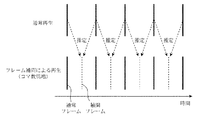•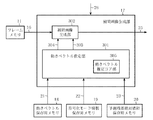••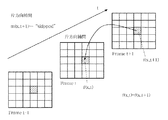•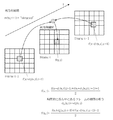•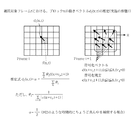•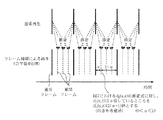•••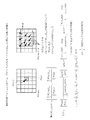••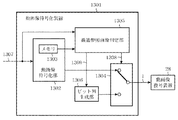•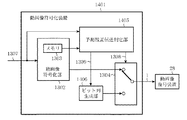•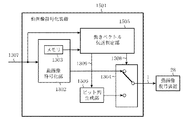•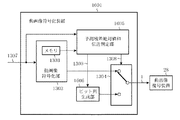•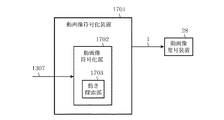•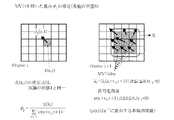•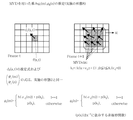•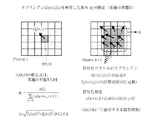•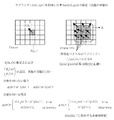••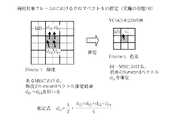•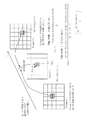•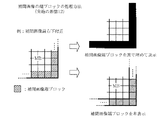•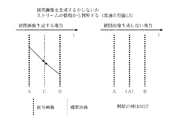•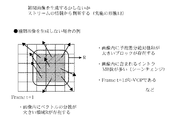•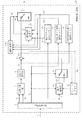•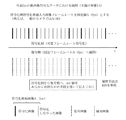## Abstract

<P>PROBLEM TO BE SOLVED: To obtain a dynamic image encoding device and a dynamic image decoding device, capable of robustly performing a frame interpolation process, without conducting much calculations anew, and further, where the threshold process is reduced, by taking out information necessary for estimating a motion vector from input stream. <P>SOLUTION: The dynamic image decoding device 28 comprises a variable length decoding unit 2, dequantization unit 7, inverse orthogonal conversion unit 8, motion compensation unit 10, frame memory 11, switch 9, adder unit 13, frame interpolation processing unit 17, memory for storing motion vector 18, memory for storing encoding mode information 19, memory for storing the sum of the absolute value of estimated residue 20 and another switch 24. <P>COPYRIGHT: (C)2007,JPO&INPIT

## Description

The present invention relates to a moving image encoding device, a moving image decoding device, and a moving image transmission system that can improve the quality of electronically converted moving images, in particular, the time resolution (number of frames / second) of moving images. Is.

Conventionally, MPEG and ITU-TH. In an international standard video coding system such as 26x, for each frame of a video signal, block data (hereinafter referred to as a macroblock) in which a luminance signal of 16 × 16 pixels and a corresponding color difference signal of 8 × 8 pixels are collected is used as a unit. A compression method based on a motion compensation technique and an orthogonal transform / transform coefficient quantization technique is employed.

Motion compensation technology is a technology that reduces the redundancy of signals in the time direction for each macroblock using high correlation existing between video frames. In the memory, a previously encoded image is used as a reference image. Accumulated and searched within the predetermined search range in the reference image for the current macroblock that is subject to motion compensation prediction and the block area with the smallest difference power, the spatial position of the current macroblock and the search result This is a technique for encoding a deviation from the spatial position of a block as a motion vector.

In many cases, the motion vector represents a local motion in each block between video frames, and research on generating an interpolation frame using the motion vector information has been made. In the simplest model, for example, when an interpolated frame is generated at an exactly middle position between temporally adjacent video frames, a value obtained by halving the value of the motion vector is used as the motion vector of the interpolated frame, There is a method of motion compensation. This model is based on the assumption that the motion between video frames is linear. The shorter the interval between video frames and the smaller the motion vector, the more the assumption is made. Is possible.

Although the motion vector often represents local motion in each block between video frames, it is actually obtained by searching for a block region with the smallest differential power, and represents local motion. Sometimes not. In such a case, the interpolated frame is disturbed and often very conspicuous. Therefore, it is a problem how to determine such a “vector not representing motion” and how to process it.

Regarding the motion vector estimation problem, to determine the reliability of a vector, the ratio of intra macroblocks in a frame, the difference between vectors with respect to adjacent macroblocks, the number of macroblocks with a large vector difference in a frame, By using the variance value of the motion vector in the frame and performing all the threshold processing, the reliability of the vector is determined with a relatively small amount of calculation. Where the amount of computation is small, it can be emphasized as an advantage, but since the appropriate threshold value changes sensitively depending on the content of the video, it is difficult to design robustly for such processing with a fixed value threshold value. (For example, refer nonpatent literature 1).

H. Sasai, S .; Kondoh and S.M. Kadono, "Frame-rate Up-conversion using Reliable Analysis of Transmitted Motion Information". IEEE International Conference on Acoustic, Speech and Signal Processing (ICASSP) 2004, ITT-L1.5, May 2004.

Since the conventional frame interpolation processing has been performed as described above, it has been difficult to perform the frame interpolation processing robustly with a small amount of calculation with respect to the problem of improving the temporal resolution of moving images.

The present invention has been made to solve the above-described problems. By extracting information necessary for motion vector estimation from an input stream (moving image encoded data), many operations are not performed again. It is another object of the present invention to obtain a moving image encoding device, a moving image decoding device, and a moving image transmission system that can perform frame interpolation processing robustly even if threshold processing is reduced.

The moving picture decoding apparatus according to the present invention analyzes moving picture coded data and extracts coding symbols in units of blocks, and restores and outputs each frame of the moving picture using the coded symbols. A decoding unit, an image generation unit that estimates an interpolation frame generation method and a motion vector of the interpolation frame based on the encoded symbol, and generates the interpolation frame; and a moving image output from the decoding unit; A switching unit that switches an output of the interpolation frame from the image generation unit at a predetermined timing.

According to the present invention, an efficient and robust frame interpolation process can be realized with a small number of processes.

Embodiment 1 FIG.
Embodiment 1 of the present invention will be described below. In the first embodiment, a video decoding device that uses each frame of video and generates an interpolated frame that temporally exists between each frame will be described. Inside the moving picture decoding apparatus, the moving picture encoded data is input, and the data is decoded / decompressed, and then subjected to frame interpolation processing using the decoded frame to generate an interpolated frame. A process for obtaining a reproduced image in a form in which the generated interpolation frame is combined with the frame is performed. Hereinafter, an image generation process corresponding to such a process is referred to as a “frame interpolation process”.

The feature of the moving picture decoding apparatus according to the first embodiment is that various information for generating an interpolation frame can be extracted from the moving picture encoded data, and no calculation is required again. FIG. 1 is a configuration diagram of a moving picture decoding apparatus according to Embodiment 1. In FIG. 1, a video decoding device 28 includes a variable length decoding unit 2, an inverse quantization unit 7, an inverse orthogonal transform unit 8, a motion compensation unit 10, a frame memory 11, a switch 9, an addition unit 13, and an interpolation image generation unit. 17, a motion vector storage memory 18, a coding mode information storage memory 19, a prediction residual absolute value sum storage memory 20, and a switch 24.

Next, the operation of the video decoding device 28 in FIG. 1 will be described. In the video decoding device 28 of FIG. 1, when the video encoded data 1 is received from the outside, entropy decoding processing is performed by the variable length decoding unit 2 (analyzing means), and the motion vector 3, encoding mode information 4, Orthogonal transform coefficient data 5, quantization step parameter 6, etc. are extracted. These extracted data are referred to as “coded symbols”.
The orthogonal transform coefficient data 5 and the quantization step parameter 6 are decoded by an inverse orthogonal transform unit 8 and an inverse quantization unit 7 (both are used as a decoding unit).

In addition, when the coding mode information 4 indicates the motion prediction mode, the switch 9 uses the motion compensation unit 10 based on the motion vector 3 and the coding mode information 4 from the reference image in the frame memory 11. While the predicted image 12 is generated and output, “0” is output when the intra mode is indicated.

In the motion prediction mode, since the output of the inverse orthogonal transform unit 8 is a prediction error signal of the motion compensation prediction result, it is generated on the encoding side (moving image encoding device) from the reference image using the motion vector 3. The same predicted image 12 is generated and added to the output 15 of the inverse orthogonal transform unit 8 in the adding unit 13 to reproduce the decoded image 14.
In the intra mode, intra-frame encoding is performed without using any reference image. In this case, since the output of the switch 9 is “0” as described above, the output 15 of the inverse orthogonal transform unit 8 becomes the decoded image 14 as it is.
The decoded image 14 is stored in the frame memory 11 to be used for generating predicted images of subsequent frames. The decoded image 14 is also output 27 to the outside of the video decoding device 28 when the switch of the switch 24 is on the upper side.

At the same time, the motion vector 3 is stored in the motion vector storage memory 18, the encoding mode information 4 is stored in the encoding mode information storage memory 19, and the absolute value sum of the output 15 of the inverse orthogonal transform unit 8 is acquired. Are stored in the prediction residual absolute value sum storage memory 20 to prepare for frame interpolation processing in the interpolated image generation unit 17.

When performing the frame interpolation process, the switch of the switch 24 is switched to the lower side at a predetermined timing 26, and the interpolated image generation unit 17 operates immediately after. When the frame interpolation processing is completed, the image output 25 of the interpolation frame is performed, and the switch of the switch 24 is returned to the upper side, so that the output 27 is output 27 from the moving image encoding device.

FIG. 2 is a diagram illustrating a case where an interpolation frame is generated at a temporally intermediate position between normal frames in the first embodiment. For example, when the interpolation frame as shown in FIG. 2 is generated, the predetermined timing 26 is a timing based on the display time of the interpolation frame generated by the upper control unit of the video decoding device 28.

Next, the interpolation image generation unit 17 will be described in detail. FIG. 3 is an internal configuration diagram of the interpolated image generation unit 17 of FIG. As illustrated in FIG. 3, the interpolation image generation unit 17 includes a motion vector estimation unit 301 and an interpolation image generation unit 302. The motion vector estimation unit 301 includes a motion vector estimation core unit 305. The interpolation image generation unit 17 divides the frame into blocks and performs processing in units of blocks. In the first embodiment, description will be made assuming that the block is 8 × 8 pixels.

FIG. 4 is a flowchart showing a processing flow of the interpolated image generation unit 17 of FIG.
FIG. 4 shows a case where the interpolation target block x is subjected to frame interpolation processing. In FIG. 4, t indicates time, and f (x, t) indicates the pixel value of the block x of Frame t. d f (x, t) is an estimated motion vector MV (forward) of block x, d b (x, t) is the estimated motion vector MV (backward), and m (x, t + 1) is an interpolation target at time “t + 1”. The encoding mode of the block x in the immediately following frame is shown. The interpolated image generation unit 17 performs the process of FIG. 4 for all the blocks in the frame to generate an interpolated frame.

Hereinafter, the operation of the motion vector estimation unit 301 in FIG. 3 will be described. The processing flow in FIG. 4 is started at a predetermined timing 26. When the processing flow is started, the motion vector estimation unit 301 acquires the encoding mode information of the calculation target block from the output 22 from the encoding mode information storage memory 19. In step ST101, it is checked whether or not the encoding mode m (x, t + 1) is “skipped”.

FIG. 5 is a diagram showing “one-way interpolation” in step ST102 of FIG. In the case of “skipped” in step ST101 of FIG. 4, since the motion of the interpolation target block x is minimal, the next interpolation image generation unit 302 performs “one-way interpolation” as shown in FIG. Accordingly, the motion vector estimation unit 301 outputs an output 303 of “one-way interpolation” and an output 304 of “motion vector (0, 0)” to the interpolation image generation unit 302.

FIG. 6 is a diagram showing “bidirectional interpolation” in step ST104 of FIG. If it is not “skipped” in step ST101 of FIG. 4, the next interpolation image generation unit 302 performs bi-directional interpolation as shown in FIG. Therefore, the motion vector estimation unit 301 outputs an output 303 of “bidirectional interpolation” to the interpolation image generation unit 302. Then, the motion vector estimation core unit 305 estimates the motion vector value of the interpolation target block, and outputs an “estimated motion vector value” 304 to the interpolation image generation unit 302. The motion vector estimation processing (step ST103) in the motion vector estimation core unit 305 will be described later.

Hereinafter, the operation of the interpolation image generation unit 302 will be described. The interpolation image generation unit 302 receives “one-way interpolation” or “two-way interpolation” as an output 303 from the motion vector estimation unit 301. In addition, a motion vector value is input at the output 304. Depending on the input data of the output 303, the operation of the interpolation image generation unit 302 is classified into the following two types.

First, the case where the output 303 is “one-way interpolation” will be described. The “one-way interpolation” shown in FIG. 5 is performed on the interpolation target block x of Frame t. FIG. 5 shows an example in which Frame t is interpolated with respect to encoded Frame t−1 and Frame t + 1. In the case of “one-way interpolation”, since the motion vector based on the output 304 is (0, 0), as shown in FIG. 5, in the encoded image of Frame t + 1, the image data of the block at the same position as the block x is obtained. To interpolate.

Next, the case where the output 303 is “bidirectional interpolation” will be described. For the interpolation target block x of Frame t, “bidirectional interpolation” shown in FIG. 6 is performed. FIG. 6 shows an example in which Frame t at an intermediate position in time is interpolated with respect to encoded Frame t−1 and Frame t + 1. The equation for interpolating the block x is given by Equation 1.

As the motion vectors d f (x, t) and d b (x, t), the motion vectors input by the output 304 are used. “Bidirectional interpolation” interpolates Frame t from both Frame t−1 and Frame t + 1. Frame t−1 uses a location shifted by d f (x, t) from the same position as the interpolation target block for interpolation. For Frame t + 1, since “d b (x, t) = − d f (x, t)” is established using the fact that Frame t is at an intermediate position in time, −d f (x , T) The shifted location is used for interpolation.

As described above, in FIG. 6, the equation for obtaining the interpolation target block is represented by Equation 2 by substituting “d b (x, t) = − d f (x, t)” into Equation 1. .

Hereinafter, the operation of the motion vector estimation core unit 305 will be described. FIG. 7 is a diagram illustrating the operation of the motion vector estimation core unit 305 of FIG. As described above, the motion vector estimation core unit 305 operates when the motion vector estimation unit 301 is not “skipped” in the encoding mode of the calculation target block, and estimates a motion vector on an interpolation frame ( (See step ST103 in FIG. 4).
Specifically, with respect to the block (calculation target block) at the same position as the interpolation target block x and the surrounding eight blocks, as indicated by the region “R” in Frame t + 1 in FIG. The motion vector on the decoded image is referred to by the output 21. Further, the encoding mode information is referred to by the output 22 from the encoding mode information storage memory 19, and the prediction residual absolute value sum is referred to by the output 23 from the prediction residual absolute value sum storage memory 20.
As shown in FIG. 7, the encoded vector (motion vector) is d (x + v i , t + 1), 0 ≦ i ≦ 8, (v 4 = 0), and the prediction residual is e (x + v i , t + 1), 0 ≦ i. When ≦ 8, (v 4 = 0), the motion vector d f (x, t) is estimated for Frame t−1 in the interpolation target block x on the interpolation frame by performing the calculation of Equation 3. Do.

And the denominator of Equation 4 is the predicted residual absolute value sum at v i .

By weighting the motion vector with the reciprocal of the prediction residual absolute value sum, a strong weight is given to the motion vector in the block having the small prediction residual absolute value sum. This is a method based on the property that the smaller the prediction residual absolute value sum is, the higher the reliability of the motion vector itself is, and the larger the prediction residual absolute value sum is, the lower the reliability of the vector itself is.

Further, since the first embodiment is an example of interpolating a frame at just an intermediate position in time as shown in FIG. 2, α = 1/2, and the motion vector d b (x, t) is given by “d b (x, t) = − d f (x, t)”.

However, among the nine calculation target blocks indicated by the region R in FIG. 7, the intra block is excluded from the calculation. The “skipped” block performs the above operation with the encoded vector (0, 0) and the prediction residual as a fixed value A. Further, even in the inter block, when there is no DCT coefficient and the sum of the absolute values of the prediction residuals is “0”, the above calculation is performed as a certain fixed value B. If all nine calculation target blocks are intra blocks, exceptionally, “d f (x, t) = (0, 0)”.

The vectors d f (x, t) and d b (x, t) estimated by the motion vector estimation core unit 305 as described above are output to the interpolated image generation unit 302.

By performing the frame interpolation processing as described above, it is possible to extract information necessary for motion vector estimation from the input stream (moving image encoded data 1), and there is no need for another calculation. The advantage is that it works robustly with almost no noise.

FIG. 8 is a diagram showing a state of frame interpolation at an arbitrary time in the first embodiment. In the first embodiment, description has been made with an example in which a frame at an intermediate position in time is interpolated as shown in FIG. 2, but for example, interpolation as shown in FIG. 8 is performed to increase the number of frames four times. It is also possible. By using the value of the relative value α as shown in FIG. 8, it is possible to generate an interpolation frame at an arbitrary place in time (scaling). When the relative time value 0 <α <1 is defined as shown in FIG. 8, in the estimation formula (Formula 3) of d f (x, t) in FIG. Then, it becomes possible to obtain d b (x, t) in a form that takes into account its direction.

Examples of applications using the moving picture decoding apparatus according to the first embodiment include portable camera moving images and DVD recorder high compression mode. In the high-compression mode of portable camera videos and DVD recorders, data is stored in a reduced number of frames and played back while performing frame interpolation processing using the method of the first embodiment. It is possible to view a video with a high time resolution.

FIG. 9 is a diagram showing a comparative example between the conventional video decoding device and the video decoding device according to the first embodiment. Comparison is made using an example in which the same video source of 30 fps is input. The upper side shows an example of moving picture output using the conventional moving picture decoding apparatus, and the lower side shows an example of moving picture output using the moving picture decoding apparatus according to the first embodiment. In the conventional example, when encoding is performed at 15 fps and the encoded data is output to the moving image decoding apparatus, 15 fps encoded data is accumulated in the moving image decoding apparatus, and reproduction is performed at 15 fps.

On the other hand, the first embodiment is an example of moving image output that is assumed to be output to the moving image decoding device 28, and at the stage of encoding by the moving image encoding device, the moving image decoding device 28 is used. Assuming that frame interpolation is performed at, in order to perform encoding with higher compression, frames are dropped and encoding is performed at 7.5 fps, and output is performed. In the video decoding device 28, encoded data of 7.5 fps is accumulated. As a result, the transmission amount and accumulation amount can be halved as compared with the conventional example, and frame interpolation processing is performed, and for example, 30 fps can be reproduced by increasing the number of frames four times.

As described above, according to the first embodiment, when the moving image decoding device 28 is used, it is possible to reduce the transmission amount and the accumulation amount as compared with the conventional case, and to reproduce a moving image with higher time resolution. Can be viewed.

The first embodiment is also applicable to MPEG-2 single field interpolation. For example, only one field can be stored as encoded data, and the remaining one field can be applied by performing interpolation using the method of the first embodiment. This will be described below with reference to FIG.

FIG. 10 is a diagram illustrating an example in which the moving picture encoding apparatus and moving picture decoding apparatus according to Embodiment 1 are applied to MPEG-2 one-field interpolation. FIG. 10 is an example in the case of outputting a certain interlaced moving image. Field extraction is performed at a stage before being input to the moving image encoding device 1201, and only the top field is input to the moving image encoding device 1201. Encode and output. The moving image decoding device 28 interpolates the Bottom Field from the decoded data of the Top Field and performs reproduction.

On the other hand, in motion picture coding such as MPEG-4, a predicted picture generated by a predicted picture generation method defined in MPEG-4 or the like in picture-type predicted picture generation using motion compensation by bidirectional prediction. Instead, the compression rate can be improved by using the interpolated frame generated in the first embodiment as a predicted image.

Embodiment 2. FIG.
The second embodiment of the present invention will be described below. In the second embodiment, the motion vector estimation core unit 305 (see FIG. 3) performs processing different from that of the first embodiment. Since other configurations are the same as those of the first embodiment, description thereof is omitted. FIG. 11 is a diagram illustrating an operation of the motion vector estimation core unit 305 of the video decoding device according to Embodiment 2.

By performing the following calculation, the motion vector d f (x, t) for Frame t−1 in the interpolation target block x on the interpolation frame is estimated.

Here, φ i (m) and φ i (n) are each expressed by Equation 6. Also, g i (m) and g i (n) are each expressed by Equation 7. In the second embodiment, h (x) is a nonlinear function that depends on x −1 , and g i (m) and g i (n) have the meanings of reciprocals of variance.

At this time, σd (m) and σd (n) represent each component of dispersion in the region R in FIG. Further, when interpolating a frame at an intermediate position just in time as shown in FIG. 2, α = ½.

Compared with the first embodiment, the second embodiment strongly weights only the calculation target block at the same position as the block x with respect to the vector of the region R, thereby reducing the variance of the motion vector of the region R. When it is small, the vector of the calculation target block is strongly weighted.

In the first embodiment, when the vector of the region R is generally small, a certain prediction residual absolute value sum in the region R is too large for a small block, and such a prediction residual absolute value sum is larger than necessary. In most cases, the motion vector of a small block is (0, 0). As a result, there are cases where there are many blocks where the estimated motion vector is zero vector more than necessary. Therefore, in the second embodiment, an algorithm using a variance value is introduced.

As described above, according to the second embodiment, the introduction of an algorithm using a variance value is a region having a motion vector with a small variance because the original motion amount is smaller than that in the first embodiment. However, there is an effect of appropriately reflecting the degree of dispersion in the estimated motion vector without dropping it to the zero vector more than necessary.

Embodiment 3 FIG.
The third embodiment of the present invention will be described below. FIG. 12 is a diagram illustrating data output from the video encoding device to the video decoding device according to Embodiment 3. The video encoding device 1201 according to Embodiment 3 assists the frame interpolation processing performed by the video decoding device 28 according to Embodiments 1 and 2 (hereinafter, interpolation assist). Specifically, the moving image encoding apparatus 1201 outputs additional information for assisting the frame interpolation process in addition to the normal encoded data 1. The part that generates the additional information is called an additional information generation unit.

The first interpolation assist (output of the reference image type in the frame interpolation process) will be described. In the frame interpolation process in the moving image decoding device 28, the moving image encoding device 1201 performs “a method of interpolating with reference to only a temporally forward frame” and “interpolating with reference to only a temporally backward frame.” Among the three types of interpolation methods “method” and “method of interpolating with reference to both frames before and after”, information on the optimum interpolation method (information indicating the optimum reference image) is individually obtained for all blocks in the frame. And output as additional information. An identification flag indicating that the frame includes the corresponding block is added before the additional information. The bit string indicating the additional information is output to the video decoding device 28 in a compressed form using, for example, arithmetic coding.

FIG. 13 is a configuration diagram of a video encoding apparatus according to Embodiment 3. Note that the moving picture coding apparatus 1301 corresponds to the moving picture coding apparatus 1201 in FIG. In FIG. 13, the moving image encoding device 1301 includes a moving image encoding unit 1302, a switch 1304, an optimum reference image determination unit 1305, and a bit string generation unit 1306. The moving image encoding unit 1302 includes a memory 1303. The optimum reference image determination unit 1305 and the bit string generation unit 1306 are additional information generation units.

Next, the operation of the moving picture coding apparatus 1301 will be described. The moving image encoding device 1301 performs encoding by input 1307 of the input image to the moving image encoding unit 1302 and then outputs 1 to the moving image decoding device 28. On the other hand, an input image 1307 is also input to the optimum reference image determination unit 1305, the encoded content is grasped by referring to the memory 1303, and an interpolated frame by the above three types of interpolation methods is provisionally generated. The input image at substantially the same time is compared for each block, and it is determined which interpolation method is optimal for each block. The determination result is input to the bit string generation unit 1306 as an output 1309, and at the same time, the switch of the switch 1304 is switched to the lower side by the output 1308. The bit string generation unit 1306 receives the determination result data at the output 1309 from the optimum reference image determination unit 1305, performs arithmetic coding on the data, and outputs the result to the video decoding device 28. When the output is finished, the switch of the switch 1304 is returned to the upper side.

As described above, according to the third embodiment, since the moving image coding apparatus 1301 has an input image, an interpolated frame is provisionally generated using the encoded data and compared with the input image. It is possible to determine an optimal interpolation method by performing this, and by outputting such an optimal interpolation method to the moving image decoding device 28 with the first interpolation assist, the image quality of the moving image subjected to frame interpolation processing There is an effect that can be expected to improve.

Embodiment 4 FIG.
The fourth embodiment of the present invention will be described below. In the fourth embodiment, the moving picture coding apparatus 1201 in FIG. 12 performs second interpolation assist (prediction residual output). If the motion decoding apparatus 28 performs normal frame interpolation processing when the motion vectors at the time of encoding are different, an interpolation frame including a block with low image quality is generated.

In Embodiment 4, in the above case, the moving picture coding apparatus 1201 outputs the prediction residual in the corresponding block on the interpolation frame in addition to the normal moving picture coded data. An identification flag indicating that the frame includes the corresponding block is added before the prediction residual data. First, the moving picture decoding apparatus 28 determines whether or not a prediction residual is output in units of frames. When outputting the prediction residual data, the block position and the prediction residual are output as additional information of the encoded data. The bit string indicating the additional information is output to the moving picture decoding apparatus 28 in a compressed form using, for example, DCT, quantization, VLC (variable length coding) used in MPEG-4.

FIG. 14 is a configuration diagram of a video encoding apparatus according to Embodiment 4. Note that the moving picture coding apparatus 1401 in FIG. 14 corresponds to the moving picture coding apparatus 1201 in FIG. In FIG. 14, the moving picture coding apparatus 1401 includes a moving picture coding unit 1302, a switch 1304, a prediction residual transmission determination unit 1405, and a bit string generation unit 1406. The moving image encoding unit 1302 includes a memory 1303. The prediction residual transmission determination unit 1405 and the bit string generation unit 1406 are additional information generation units.

Next, the operation of the moving image encoding device 1401 will be described. The video encoding device 1301 performs encoding by input 1307 of the input image to the video encoding unit 1302, and then outputs the video encoded data 1 to the video decoding device 28. On the other hand, an input image 1307 is also input to the prediction residual transmission determination unit 1405, the encoded contents are grasped by referring to the memory 1303, an interpolation frame is provisionally generated, and an input image at approximately the same time as the interpolated frame. And for each block, it is determined whether it is necessary to send a prediction residual for each block. As a result, if it is necessary to send the prediction residual even in one block, the block position and the prediction residual are transmitted to the bit string generation unit 1406 at the output 1309, and at the same time, the switch of the switch 1304 is switched to the lower side via the output 1308. The bit string generation unit 1406 receives the data (block position and prediction residual) from the prediction residual transmission determination unit 1405 at the output 1309, performs appropriate compression on the data, and outputs the data to the video decoding device 28. When the output is finished, the switch of the switch 1304 is returned to the upper side.

As described above, according to the fourth embodiment, since there is an input image in the moving image encoding apparatus, an interpolation frame is provisionally generated using the encoded data and compared with the input image. Thus, it is possible to examine an interpolation frame including a block with remarkably low image quality, and by outputting the prediction residual in the corresponding block on the interpolation frame to the moving image decoding device 28 by such second interpolation assist. There is an effect that the image quality of the frame subjected to the frame interpolation process can be expected to be improved.

Embodiment 5. FIG.
The fifth embodiment of the present invention will be described below. In the fifth embodiment, the moving picture coding apparatus 1201 in FIG. 12 performs the third interpolation assist (output of the motion vector on the interpolation frame). When the motion vector at the time of encoding is different and the moving image decoding apparatus 1 performs the processing of the normal motion vector estimation core unit 305 (step ST103 in FIG. 4) as shown in the first and second embodiments. This results in an interpolated frame including blocks with low image quality. In particular, in the case of an intra block, since the motion vector itself does not exist in the encoded data, such a state is likely to occur.

In the fifth embodiment, in the above case, the moving image encoding apparatus 1201 outputs the motion vector in the corresponding block on the interpolation frame as additional information in addition to the normal encoded data. An identification flag indicating that the frame includes the corresponding block is added in front of the motion vector data. The video decoding device 28 first determines whether or not a motion vector is output in units of frames. When outputting motion vector data, the corresponding block position and motion vector are output as additional information.

FIG. 15 is a configuration diagram of a video encoding apparatus according to Embodiment 5. Note that the moving picture coding apparatus 1501 in FIG. 15 corresponds to the moving picture coding apparatus 1201 in FIG. In FIG. 15, the moving picture coding apparatus 1501 includes a moving picture coding unit 1302, a switch 1304, a motion vector transmission determination unit 1505, and a bit string generation unit 1506. The moving image encoding unit 1302 includes a memory 1303. The motion vector transmission determination unit 1505 and the bit string generation unit 1506 are additional information generation units.

Next, the operation of the moving picture coding apparatus 1501 will be described. The video encoding device 1301 performs encoding by input 1307 of the input image to the video encoding unit 1302, and then outputs the video encoded data 1 to the video decoding device 28. On the other hand, an input image 1307 is also input to the motion vector transmission determination unit 1505, the encoded contents are grasped by referring to the memory 1303, an interpolated frame is temporarily generated, and an input image at substantially the same time as the interpolated frame Are compared for each block, and it is determined whether or not the image quality is significantly deteriorated. If there is a block with low image quality, it is determined whether the motion vector needs to be sent by determining whether the image quality is improved by changing the motion vector on the interpolation frame. As a result, if it is necessary to send a motion vector even in one block, the block position and the motion vector value to be transmitted are transmitted to the bit string generation unit 1506 at the output 1309, and at the same time, the switch of the switch 1304 is switched to the lower side via the output 1308. . The bit string generation unit 1506 receives data from the motion vector transmission determination unit 1505 via 1309, performs appropriate compression on the data, and outputs the data to the video decoding device 28. When the output is finished, the switch of the switch 1304 is returned to the upper side.

As described above, according to the fifth embodiment, since the moving image encoding apparatus has an input image, an interpolation frame is temporarily generated using the encoded data and compared with the input image. Therefore, since the normal motion vector estimation core unit process can examine an interpolation frame including a block with extremely low image quality, the third interpolation assist can be used to check the interpolation frame in the corresponding block on the interpolation frame. By outputting the optimal motion vector, it is possible to expect an improvement in the image quality of the frame subjected to the frame interpolation process.

Embodiment 6 FIG.
The sixth embodiment of the present invention will be described below. In the sixth embodiment, the moving picture coding apparatus 1201 in FIG. 12 performs the fourth interpolation assist (output of the prediction residual absolute value sum). In coding for real video, generally, the prediction residual absolute value sum hardly becomes zero. However, if there is a block without a DCT coefficient, in the video decoding device 28, the prediction residual absolute value sum in that block becomes zero.

In the sixth embodiment, in the above case, the moving image coding apparatus 1201 outputs the prediction residual absolute value sum only for the corresponding block in addition to the normal coded data. An identification flag indicating that the frame includes the corresponding block is added before the prediction residual absolute value sum data. The moving picture decoding device 28 first determines whether or not the prediction residual absolute value sum is output for each frame. When outputting the prediction residual absolute value sum data, the corresponding block position and the prediction residual absolute value sum are output as additional information. The bit string indicating the additional information is compressed by, for example, arithmetic coding or the like, and is output to the video decoding device 28 in a form converted into a very short code.

FIG. 16 is a configuration diagram of a video encoding apparatus according to Embodiment 6. Note that the moving picture coding apparatus 1601 in FIG. 16 corresponds to the moving picture coding apparatus 1201 in FIG. In FIG. 16, the moving image encoding device 1601 includes a moving image encoding unit 1302, a switch 1304, a prediction residual absolute value sum transmission determination unit 1605, and a bit string generation unit 1606. The moving image encoding unit 1302 includes a memory 1303. The prediction residual absolute value sum transmission determination unit 1605 and the bit string generation unit 1506 are additional information generation units.

Next, the operation of the moving image encoding device 1601 will be described. The video encoding device 1301 performs encoding by input 1307 of the input image to the video encoding unit 1302, and then outputs the video encoded data 1 to the video decoding device 28. On the other hand, the input 1307 of the input image is also input to the prediction residual absolute value sum transmission determination unit 1605, the encoded contents are grasped by referring to the memory 1303, an interpolated frame is provisionally generated, and approximately the same time as the interpolated frame. Are compared for each block, and it is determined whether or not the image quality is significantly deteriorated. If there is a block with poor image quality, determine whether it is necessary to send the sum of the predicted residual absolute values by determining whether the image quality is improved by correcting the predicted residual absolute value sum on the interpolation frame To do. As a result, if it is necessary to send the prediction residual absolute value sum even for one block, the block position and the motion vector value to be output are transmitted to the bit string generation unit 1606 at the output 1309, and at the same time, the switch 1304 is switched off at the output 1308. Switch to the side. The bit string generation unit 1606 receives data from the prediction residual absolute value sum transmission determination unit 1605 at the output 1309, performs appropriate compression on the data, and outputs the data to the video decoding device 28. When the output is finished, the switch of the switch 1304 is returned to the upper side.

As described above, according to the sixth embodiment, since the moving image encoding apparatus 1601 has an input image, an interpolated frame is provisionally generated using the encoded data and compared with the input image. By doing so, it is possible to examine an interpolation frame including a block with extremely low image quality in the processing of the normal motion vector estimation core unit. Therefore, with such fourth interpolation assist, the prediction remaining in the corresponding block on the interpolation frame can be checked. By outputting the sum of absolute differences, there is an effect that the image quality of the frame subjected to the frame interpolation process can be expected.

When the first to fourth interpolation assists described in the third to sixth embodiments are performed, the additional information type is output by adding a type flag indicating the type of additional information to the front of the additional information. Means.

Embodiment 7 FIG.
The seventh embodiment of the present invention will be described below. In the seventh embodiment, the moving picture coding apparatus 1201 of FIG. 12 performs the fifth interpolation assist (limitation of motion vectors on the coded picture). When encoding, the moving image encoding device 1201 does not use a motion vector that references the outside of the frame.

FIG. 17 is a configuration diagram of a video encoding apparatus according to Embodiment 7. Note that the video encoding device 1701 in FIG. 17 corresponds to the video encoding device 1201 in FIG. The moving picture coding apparatus 1701 in FIG. 17 includes a moving picture coding unit 1702. In addition, the moving image encoding unit 1702 includes a motion search unit 1703.

Next, the operation of the moving image encoding unit 1701 will be described. The video encoding device 1701 performs encoding by input 1307 of the input image to the video encoding unit 1702, and then outputs the video encoded data 1 to the video decoding device 28. In the encoding process in the moving image encoding unit 1702, the motion search unit 1703 performs a motion search so as not to generate a motion vector that refers to the outside of the frame, and generates the encoded moving image data 1.

As described above, according to the seventh embodiment, it is possible to secure at least one of the reference images in the frame interpolation process by performing encoding so as not to use the motion vector that refers to the outside of the frame. There is.

Embodiment 8 FIG.
The eighth embodiment of the present invention will be described below. In the eighth embodiment, another process of the motion vector estimation core unit 305 in the first and second embodiments will be described. Since other configurations are the same as those of the first embodiment, description thereof is omitted. Details are shown in FIGS. 18 and 19, respectively.

First, a process different from the process of the motion vector estimation core unit 305 in the first embodiment (the process in the eighth embodiment) will be described. FIG. 18 is an explanatory diagram 1 of weight estimation using MVD in the eighth embodiment.
In FIG. 18, the calculation formula of the reference vector d f (x, t) with respect to Frame t−1 in the processing target block x on the interpolation image (interpolation frame) is the same as Formula 3 shown in the first embodiment. is there. The calculation formula of the weight φ i in Formula 3 is different from that in Embodiment 1 (Formula 4), and the calculation is performed by the calculation formula as Formula 9 below.

The denominator of Equation 9 is the same as the denominator of Equation 4, and represents the predicted residual absolute value sum in v i . The numerator part p (k i ) is different from the numerator of Equation 4, p (x) is a non-linear function that depends on x −1 , and k i is an MVD (Motion Vector Difference): motion vector difference value ) Vector size. portion of p (k i) as a whole represents the inverse of the magnitude of the MVD.

p (x) is a non-linear function that depends on x −1 , but in actual applications, the amount of division may be large, so x and p (x) are made to correspond one-to-one. It is also conceivable to calculate p (x) from x by looking up the table.

The magnitude of the MVD represents the degree of isolation of the vector, and the higher the MVD, the higher the possibility of the degree of isolation. Therefore, by incorporating the reciprocal of the size of the MVD in the calculation formula (equation 9) of the weight φ i This has the effect of reducing the vector weight. The reason is that the isolated vector has a high probability of low reliability. By reducing the weight of an isolated vector, there is an effect of improving the vector estimation accuracy.

In the case of MPEG-4, for example, the value described in the encoded data can be used as it is in the case of MVD, so that the amount of calculation can be reduced.

This is the description of the other processing of the motion vector estimation core unit 305 in the first embodiment.
Next, another process (the process of the eighth embodiment) of the motion vector estimation core unit 305 in the second embodiment will be described. FIG. 19 is an explanatory diagram 2 of weight estimation using MVD in the eighth embodiment.

In FIG. 19, the calculation formula of the reference vector d f (x, t) for Frame t−1 in the processing target block x on the interpolation image is the same as Formula 5 shown in the second embodiment. Furthermore, the calculation formulas of the weights φ i (m) and φ i (n) in Formula 5 are the same as those in Formula 6 shown in the second embodiment. Unlike the second embodiment (Equation 7), the calculation equations of g i (m) and g i (n) in Equation 6 are performed as shown in Equation 10 below.

In Expression 10, h (σ d (m)) and h (σ d (n)) have the same meaning as in the second embodiment and are factors depending on the reciprocal of the variance. p (k i) has the same meaning as Equation 9, a factor which depends on the reciprocal of the size of the MVD. In an actual application, the calculation amount of division may be large. Therefore, it is possible to calculate h (x) from x by looking up a table in which x and h (x) correspond one-to-one. It is conceivable that g i (x) itself is directly looked up by switching the table in accordance with the value of i .

By adding the MVD factor to the second embodiment, there is an effect of improving the estimation accuracy of the reference vector d f (x, t) more than adding the MVD factor to the first embodiment.

As described above, according to the eighth embodiment, the estimation accuracy of the reference vector d f (x, t) is further improved by adding the MVD factor to the first or second embodiment. Can be made.
Also, for MVD, depending on the standard adopted, the numerical value in the encoded data can be used as it is, which has the effect of reducing the amount of calculation.

Embodiment 9 FIG.
The ninth embodiment of the present invention will be described below. In the ninth embodiment, another process of the motion vector estimation core unit 305 in the first and second embodiments will be described. Since other configurations are the same as those of the first embodiment, description thereof is omitted. Details are shown in FIGS. 20 and 21, respectively.

First, another process of the motion vector estimation core unit 305 in the first embodiment will be described. FIG. 20 is an explanatory diagram 1 of weight estimation using a Laplacian value in the ninth embodiment.
In FIG. 20, the calculation formula of the reference vector d f (x, t) with respect to Frame t−1 in the processing corresponding block on the interpolated image is the same as Formula 3 shown in the first embodiment. The calculation formula of the weight φ i in Formula 3 is different from that in Embodiment 1 (Formula 4), and the calculation is performed by the calculation formula as Formula 11 below.

The denominator of Expression 11 is the same as the denominator of Expression 4, and represents the sum of absolute values of prediction residuals in v i . The part of the molecule z (l i ) is different from the numerator of Equation 4, z (x) is a nonlinear function that depends on x −1 , and l i is a Laplacian expressed by Equation 12 below. The magnitude of the value. The portion of z (l i ) represents the reciprocal of the Laplacian value as a whole.

Although z (x) is a non-linear function that depends on x −1 , in some practical applications, the amount of division may be large, so x and z (x) are made to correspond one-to-one. It is also conceivable to calculate z (x) from x by looking up the table.

The size of the Laplacian value represents the isolation of the vector, since a large isolated degree higher the Laplacian value, by incorporating the size inverse of the Laplacian value to the formula of the thus weighted phi i, the isolated vector There is an effect of reducing the weight. The reason is that the isolated vector has a high probability of low reliability. By reducing the weight of an isolated vector, there is an effect of improving the vector estimation accuracy.

The difference between the MVD in the eighth embodiment and the Laplacian value in the ninth embodiment is that the Laplacian value has a larger calculation amount than the MVD, but the Laplacian value has an effect that the estimation accuracy is improved more than the MVD. .

Details of the calculation method of l i (m) and l i (n), which are components of the Laplacian value, are described in FIG. FIG. 22 is an explanatory diagram of a Laplacian value calculation method according to the ninth embodiment. With respect to l 4 (m) and l 4 (n), which are Laplacian values of the central block in the region R in FIG.

Then, with respect to all the regions R in the image, the Laplacian values of all the blocks are calculated by performing the calculation of Equation 13, so that l i (m), l i (n) (0 ≦ i ≦ 8) can be calculated.

This is the description of the other processing of the motion vector estimation core unit 305 in the first embodiment.
Next, another process of the motion vector estimation core unit 305 in the second embodiment will be described. FIG. 21 is an explanatory diagram 1 of weight estimation using a Laplacian value in the ninth embodiment.

In FIG. 21, the calculation formula of the reference vector d f (x, t) for Frame t−1 in the processing block on the interpolation image is the same as Formula 5 shown in the second embodiment. Furthermore, the calculation formulas of the weights φ i (m) and φ i (n) in Formula 5 are the same as those in Formula 6 shown in the second embodiment. The calculation formulas g i (m) and g i (n) in Formula 6 are different from those in Embodiment 2 (Formula 7), and are performed as shown in Formula 14 and Formula 15 below.

Expression 14 is a case where dispersion is not used, and Expression 15 is a case where dispersion is used. In Expression 15, h (σ d (m)) and h (σ d (n)) have the same meaning as in the second embodiment and are factors depending on the reciprocal of the variance. z (| l i (m) |) and z (| l i (n) |) have the same meaning as z (l i ) in Equation 13, and are factors that depend on the inverse of the Laplacian value. . In an actual application, the calculation amount of division may be large. Therefore, it is possible to calculate h (x) from x by looking up a table in which x and h (x) correspond one-to-one. It is conceivable that g i (x) itself is directly looked up by switching the table in accordance with the value of i .

In contrast to the method that also uses the variance shown in the second embodiment, the Laplacian value is compared to the method that does not use the variance as in the first embodiment by performing a calculation like Equation 15 that further considers the factor of the Laplacian value. There is an effect that the estimation accuracy of the reference vector d f (x, t) is further improved compared with the above factor.

In addition, a method of performing a weighting operation according to the complexity of each block is also conceivable. This is based on the fact that the higher the block complexity, the greater the reliability of the vector. For example, the complexity is a dispersion value of the luminance value of the pixel, and the weight is increased as the complexity increases.

As described above, according to the ninth embodiment, the estimation accuracy of the reference vector d f (x, t) is further increased by adding the factor of the Laplacian value to the first or second embodiment. Can be improved.

Embodiment 10 FIG.
The tenth embodiment of the present invention will be described below. In the tenth embodiment, a method of performing color difference vector estimation using the luminance vector estimation results calculated in the first embodiment, the second embodiment, the eighth embodiment, and the ninth embodiment will be described. Details are shown in FIG. FIG. 23 is an explanatory diagram of estimation of a color difference vector in the tenth embodiment.

In FIG. 23, the estimation results of the luminance vector of each block in a certain macroblock obtained in the first embodiment, the second embodiment, the eighth embodiment, and the ninth embodiment are d f1 to d f4 , respectively. Using the result, the color difference vector d fc of the macroblock is calculated as in Expression 16.

As described above, according to the tenth embodiment, it is possible to colorize an interpolation image by estimating a color difference vector from a luminance vector estimation result.

Embodiment 11 FIG.
The eleventh embodiment of the present invention will be described below. In the eleventh embodiment, another process in step ST104 of FIG. 4 in the first embodiment will be described. Since other configurations are the same as those of the first embodiment, description thereof is omitted. Details are shown in FIG. FIG. 24 is an explanatory diagram of interpolation weighting using quantization coefficient values in the eleventh embodiment.

The process of step ST104 in the first embodiment is a simple addition average represented by Equation 1 and Equation 2, but in this embodiment, the average value of the quantization coefficients of all macroblocks in the decoded image is used. Add weight. The average value of the quantization coefficient in Frame t−1 is q (t−1), the average value of the quantization coefficient in Frame t + 1 is q (t + 1), and weighting calculation is performed to obtain Equation (17).

As described above, according to the eleventh embodiment, by performing the weighting calculation as in Expression 17, a larger weight is applied to an image with a small quantization coefficient, that is, a decoded image with good image quality. Also has the effect of improving image quality.

Embodiment 12 FIG.
The twelfth embodiment of the present invention will be described below. In the twelfth embodiment, description will be made regarding processing of an end block in an interpolation frame. An overview is shown in FIG. FIG. 25 is an explanatory diagram of a method for processing a block located at an end according to the twelfth embodiment.

The end block depends on the estimated luminance vector. However, when bi-directional interpolation is performed, one of the vectors often points outside the screen, which may cause deterioration in image quality.
When one of the vectors points outside the screen, a method of performing one-way interpolation using only the estimated vector pointing inside the screen is conceivable, but there may be a case where no vector pointing inside the screen exists.

Therefore, as shown in FIG. 25, there is a method in which the block located at the edge of the screen is not interpolated and is filled with black immediately before display, or the block located at the edge itself is not displayed. It is done. In addition, it is possible to consider a method of drawing a frame line with a certain predetermined color after the block located at the end is not displayed.

As described above, according to the twelfth embodiment, processing the block located at the end as shown in FIG. 25 has an effect of improving the image quality although the substantial resolution is reduced.

Embodiment 13 FIG.
The thirteenth embodiment of the present invention will be described below. In the thirteenth embodiment, a method for determining in advance whether or not to create an interpolation frame from information of moving image encoded data and a process when no interpolation is performed will be described. Details are described in FIGS. 26, 27, and 28.

FIG. 26 is an explanatory diagram of the case where the interpolation image is generated and not generated in the thirteenth embodiment. FIG. 26 shows a case where an interpolated image is generated and a case where it is not generated based on information of moving image encoded data (input stream).

When it is determined that the interpolation is performed, vector estimation as in the first, second, eighth, and ninth embodiments is performed as shown on the left side of FIG. 26, an interpolation frame is created, and the interpolation frame is displayed.

On the other hand, if it is determined not to interpolate, as shown on the right side of FIG. 26, the interpolated frame is not created and the frame t-1 image is displayed as it is as Frame t, or the frame t-1 image is displayed. There is a method of displaying as it is and not updating the display until Frame t + 1.

FIG. 27 is an explanatory diagram of determination materials when an interpolation image is not generated in the thirteenth embodiment. FIG. 27 shows conditions when an interpolation image is not generated. For example, as shown in the figure on the left, if there is even one region R with a large vector variance in the image, the image quality in the vicinity of that region of the interpolation frame may be significantly reduced, and no interpolation is performed. Judge.

In addition, when there is a block having a large prediction difference absolute value sum, the prediction difference absolute value sum is large even though motion search (ME) is performed on the encoding side. Since there is no similar image in the vicinity of the area, the image quality in the area may be significantly deteriorated. Therefore, it is determined that no interpolation is performed.

As another example, for example, when there are many intra MBs in the decoded image, such as a scene change, or when Frame t + 1 is I-VOP (Video Object Plane), it is determined that no interpolation is performed.

FIG. 28 is a block diagram of a moving picture decoding apparatus in which an interpolation control unit is added as a constituent element in the thirteenth embodiment. The block diagram of FIG. 28 is a video decoding device in which an interpolation control unit 11201 is added to the block diagram of the video decoding device shown in FIG. The interpolation control unit 11201 determines whether to interpolate or not interpolate as shown in FIG. 27 with reference to the motion vector storage memory 18, the coding mode information storage memory 19, and the prediction residual absolute value sum storage memory 20. The switch 24 is controlled by controlling the timing 26.

As described above, according to the thirteenth embodiment, by determining each time whether or not to interpolate, if it is determined not to interpolate, the image quality can be significantly lowered when interpolation is performed. As a result, there is an effect that the total image quality is improved.

Embodiment 14 FIG.
The fourteenth embodiment of the present invention will be described below. In the fourteenth embodiment, an interpolation method for variable frame rate moving image encoded data is described. An outline is shown in FIG. FIG. 29 is an explanatory diagram of an interpolation method for variable frame rate moving image encoded data according to the fourteenth embodiment.

As an operation of a general encoding device, it is common to create moving image encoded data having a variable frame rate in order to fit the data amount in a band due to a limitation of a transmission band. As a method of performing interpolation for such variable frame rate moving image encoded data, there is a method in which the value A (display rate information) shown in FIG. 29 is sent from the encoding side to the decoding side in advance.
Also, by predetermining the value of A as the entire system including the encoding side and the decoding side, both the moving image encoding device side and the moving image decoding device side are known, and then the decoding side determines that A There is a method of performing interpolation so as to be fps.
Alternatively, as another method for sharing the value of A between the encoding side and the decoding side, for example, the value of A is stored in an area such as user_data in the moving image encoded data, and the encoding side transfers to the decoding side. There is also a method of sending the value of A.
The moving image encoding apparatus side includes an encoded image selection unit that selects a frame image to be actually encoded as necessary.
The moving picture decoding apparatus side includes display rate decoding means for decoding display rate information from the moving picture encoding apparatus side as necessary.

For example, when a normal camera is used on the encoding side, the value of A is A = 30 if all images input from the camera to the moving image encoding apparatus are candidates for the encoded image. Become.
Also, for example, if the system originally has a maximum frame rate of 10 fps with a fixed frame interval, A = 10 may be considered.

As an interpolation method, it is possible to perform interpolation by changing the value of α in the form described in FIG. 8 of the first embodiment.

As described above, according to the fourteenth embodiment, by interpolating variable frame rate moving image encoded data to a fixed frame rate, compared to a method of simply interpolating between frames, There is an effect that the movement becomes smoother.

1 is a configuration diagram of a moving picture decoding apparatus according to Embodiment 1. FIG. In Embodiment 1, it is a figure which shows the case where an interpolation frame is produced | generated in the temporal intermediate position of normal frames. It is an internal block diagram of the interpolation image generation part of FIG. It is the flowchart which showed the processing flow of the interpolation image generation part of FIG. It is a figure which shows "one-way interpolation" in step ST102 of FIG. It is a figure which shows "bidirectional interpolation" in step ST104 of FIG. It is the figure which showed operation | movement of the motion vector estimation core part of FIG. 6 is a diagram showing a state of frame interpolation at an arbitrary time in Embodiment 1. FIG. It is the figure which showed the comparative example of the conventional moving image decoding apparatus and the moving image decoding apparatus which concerns on Embodiment 1. FIG. It is a figure which shows the example which applied the moving image encoding apparatus and moving image decoding apparatus which concern on Embodiment 1 to the one-field interpolation of MPEG-2. FIG. 11 is a diagram illustrating an operation of a motion vector estimation core unit of the video decoding device according to Embodiment 2. It is a figure which shows the data output from the moving image encoding apparatus which concerns on Embodiment 3 to a moving image decoding apparatus. [Fig. 10] Fig. 10 is a configuration diagram of a video encoding device according to Embodiment 3. [Fig. 10] Fig. 10 is a configuration diagram of a moving image encoding device according to Embodiment 4. [Fig. 10] Fig. 10 is a configuration diagram of a moving image encoding device according to Embodiment 5. FIG. 10 is a configuration diagram of a video encoding apparatus according to Embodiment 6. FIG. 10 is a configuration diagram of a video encoding device according to Embodiment 7. FIG. 10 is an explanatory diagram 1 of weight estimation using MVD in an eighth embodiment. FIG. 10 is an explanatory diagram 2 of weight estimation using MVD in the eighth embodiment. FIG. 10 is an explanatory diagram 1 of weight estimation using a Laplacian value in the ninth embodiment. FIG. 20 is an explanatory diagram 2 of weight estimation using a Laplacian value in the ninth embodiment. FIG. 20 is an explanatory diagram of a Laplacian value calculation method according to the ninth embodiment. FIG. 20 is an explanatory diagram of color difference vector estimation in the tenth embodiment. FIG. 38 is an explanatory diagram of interpolation weighting using quantization coefficient values in the eleventh embodiment. 218 is an explanatory diagram of a processing method of a block positioned at an end in Embodiment 12. [FIG. It is explanatory drawing when not producing | generating the interpolation image in Embodiment 13. FIG. FIG. 38 is an explanatory diagram of determination materials when an interpolation image is not generated in the thirteenth embodiment. [Fig. 38] Fig. 38 is a block diagram of a video decoding device in which an interpolation control unit is added as a component in Embodiment 13. FIG. 38 is an explanatory diagram of an interpolation method for variable frame rate moving image encoded data according to the fourteenth embodiment.

Explanation of symbols

DESCRIPTION OF SYMBOLS 1 Video coding data, 2 Variable length decoding part, 3 Motion vector, 4 Coding mode information, 5 Orthogonal transformation coefficient data, 6 Quantization step parameter, 7 Inverse quantization part, 8 Inverse orthogonal transformation part, 9 Switch 10 motion compensation unit, 11 frame memory, 12 predicted image, 13 addition unit, 14 decoded image, 15 output, 16 output, 17 interpolated image generation unit, 18 motion vector storage memory, 19 encoding mode information storage memory, 20 Prediction residual absolute value sum storage memory, 21 output, 22 output, 23 output, 24 switch, 25 image output, 26 timing, 27 output, 28 video decoding device, 301 motion vector estimation unit, 302 interpolated image generation Unit, 303 output, 304 output, 305 motion vector estimation core unit, 1301 moving image encoding device, 1302 moving image encoding unit, 1303 1304 switching unit, 1305 optimal reference image determination unit, 1306 bit string generation unit, 1307 input, 1308 output, 1309 output, 1401 moving image encoding device, 1405 prediction residual transmission determination unit, 1406 bit string generation unit, 1501 moving image Coding apparatus, 1505 motion vector transmission determination unit, 1506 bit string generation unit, 1601 video encoding apparatus, 1605 prediction residual absolute value sum transmission determination unit, 1606 bit string generation unit, 1701 video encoding apparatus, 1702 video code Conversion unit, 1703 motion search unit, 11201 interpolation control unit.

## Claims (22)

1. Analysis means for analyzing moving image encoded data and extracting encoded symbols in units of blocks;
Decoding means for restoring and outputting each frame of the moving image using the encoded symbol;
An image generation unit that generates an interpolation frame by estimating an interpolation frame generation method and an interpolation frame motion vector based on the encoded symbol;
A moving picture decoding apparatus comprising: a switching unit that switches a moving picture output from the decoding unit and an interpolation frame output from the image generation unit according to a predetermined rule.
2. The encoded symbol includes a motion vector, encoding mode information, and a prediction residual;
The image generator
A first step of selecting at least one moving image frame as a reference image from among the moving image frames obtained from the decoding unit according to the encoding mode information;
In the calculation target block on the reference image and its neighboring blocks, the reciprocal of the prediction residual is used as a weight, the weighted average calculation for each component of the motion vector is performed, and scaling is performed according to the temporal position of the interpolation frame. Performing a second step of estimating a motion vector of the interpolated frame indicating a reference position of the reference image;
The moving image decoding apparatus according to claim 1, further comprising: a third step of generating the interpolation frame using a pixel at a reference position of the reference image based on the reference vector.
3. In the second step,
A variance value of a motion vector is calculated in the calculation target block on the reference image and its neighboring blocks, and for the calculation target block, a product of the reciprocal of the prediction residual and the reciprocal of the variance value is used as a weight, For the neighboring blocks, the inverse of the prediction residual is used as a weight, weighted average calculation is performed for each component of the motion vector, and scaling is performed according to the temporal position of the interpolated frame, so that the reference image 3. The moving picture decoding apparatus according to claim 2, wherein a motion vector of the interpolation frame indicating a reference position is estimated.
4. A motion vector storage memory for storing the motion vector;
A memory for storing encoding mode information for storing the encoding mode information;
4. The moving picture decoding apparatus according to claim 2, further comprising a prediction residual absolute value sum storage memory for storing the prediction residual.
5. First analysis means for analyzing moving image encoded data and extracting encoded symbols in units of blocks;
Second analysis means for analyzing data including information for assisting the image generation unit;
Decoding means for restoring and outputting each frame of the moving image using the encoded symbol;
An image generation unit that generates an interpolation frame by estimating an interpolation frame generation method and an interpolation frame motion vector based on the encoded symbol;
A moving picture decoding apparatus comprising: a switching unit that switches a moving picture output from the decoding unit and an interpolation frame output from the image generation unit according to a predetermined rule.
6. A moving image encoding unit that encodes a moving image to generate moving image encoded data;
An additional information generating unit that compares the input image and the temporarily generated interpolation frame for each block and generates additional information for assisting the frame interpolation processing;
A moving picture coding apparatus that outputs the moving picture coded data and the additional information.
7.   7. The moving picture encoding apparatus according to claim 6, wherein an identification flag indicating presence / absence of the additional information is added to the additional information and output.
8.   8. The moving picture encoding apparatus according to claim 6, wherein a type flag indicating a type of the additional information is added to the additional information and output.
9.   9. The moving image encoding apparatus according to claim 6, wherein the additional information includes data indicating a reference image that is optimal when generating an interpolation frame.
10.   The moving image encoding apparatus according to claim 6, wherein the additional information includes data indicating a block position of a block and a prediction residual in the interpolation frame.
11.   9. The moving picture encoding apparatus according to claim 6, wherein the additional information includes data indicating a block position and a motion vector of the block in the interpolation frame.
12.   The moving image code according to any one of claims 6 to 8, wherein the additional information includes data indicating a block position of the block in the interpolation frame and a predicted residual absolute value sum. Device.
13.   The said moving image encoding part is provided with the motion search part which performs a motion search so that the motion vector which refers the out-of-frame may not be generated, The any one of Claims 6-12 characterized by the above-mentioned. Video encoding device.
14. A video decoding device according to claim 5;
A moving image encoding device according to claim 6,
The moving image encoding apparatus outputs the moving image encoded data with information for assisting the image generating unit added thereto, and the moving image decoding apparatus inputs the moving image encoded data and the additional information. Then, the second information analyzing unit analyzes the additional information to perform image generation processing in cooperation with each other.
15. The encoded symbol includes a motion vector difference value;
In the second step,
In the calculation target block on the reference image and the peripheral blocks, the product of the reciprocal of the motion vector difference value and the reciprocal of the prediction residual is used as a weight, and the weighted addition average calculation for each component of the motion vector is performed. 3. The moving picture decoding apparatus according to claim 2, wherein the motion vector of the interpolation frame indicating the reference position of the reference image is estimated by performing scaling according to the temporal position of the interpolation frame.
16. In the second step,
In the calculation target block on the reference image and its neighboring blocks, the product of the reciprocal of the calculated Laplacian value of the motion vector and the reciprocal of the prediction residual is used as a weight, and the weighted average calculation for each component of the motion vector is performed. 3. The moving picture decoding apparatus according to claim 2, wherein a motion vector of the interpolation frame indicating a reference position of the reference image is estimated by performing scaling according to a temporal position of the interpolation frame. .
17. In the third step,
By calculating the average value of the quantization coefficient of the reference image, using the pixel at the reference position of the reference image by the reference vector, and performing weighted addition of the pixel by the average value of the quantization coefficient, The moving image decoding apparatus according to claim 2, wherein the interpolation frame is generated.
18. In the third step,
Based on the reference vector, the interpolation frame is calculated using the pixels at the reference position of the reference image, and all the blocks located at the end of the calculated interpolation frame are filled with a specific color and are generated as the interpolation frame. The moving picture decoding apparatus according to claim 2.
19. The predetermined rule is a rule depending on a predetermined time interval,
2. The moving image decoding apparatus according to claim 1, wherein the moving image output from the decoding unit and the output of the interpolation frame from the image generation unit are switched.
20. The predetermined rule is a rule depending on the encoded symbol,
The moving image according to claim 1, further comprising an interpolation control unit that determines switching between output of a moving image from the decoding unit and output of an interpolation frame from the image generation unit using the encoded symbol. Image decoding device.
21. Encoded image selection means for selecting a frame image to be actually encoded for each frame image of a moving image input according to a predetermined display rate,
Only for the frame image selected by the encoded image selection means, moving image encoded data is generated, and display rate information of the input moving image signal is multiplexed and transmitted to the moving image encoded data. A video encoding device.
22. Display rate decoding means for decoding display rate information included in the moving image encoded data,
The output of the moving image from the said decoding means and the output of the interpolation frame from the said image generation part are switched based on the said display rate information decoded by the said display rate decoding means. Video decoding device.
JP2005306267A 2005-03-04 2005-10-20 Dynamic image encoding device, dynamic image decoding device and dynamic image transmitting system Pending JP2006279917A (en)

## Priority Applications (2)

Application Number Priority Date Filing Date Title
JP2005061056 2005-03-04
JP2005306267A JP2006279917A (en) 2005-03-04 2005-10-20 Dynamic image encoding device, dynamic image decoding device and dynamic image transmitting system

## Applications Claiming Priority (1)

Application Number Priority Date Filing Date Title
JP2005306267A JP2006279917A (en) 2005-03-04 2005-10-20 Dynamic image encoding device, dynamic image decoding device and dynamic image transmitting system

## Publications (1)

Publication Number Publication Date
JP2006279917A true JP2006279917A (en) 2006-10-12

# Family

## Family Applications (1)

Application Number Title Priority Date Filing Date
JP2005306267A Pending JP2006279917A (en) 2005-03-04 2005-10-20 Dynamic image encoding device, dynamic image decoding device and dynamic image transmitting system

## Country Status (1)

JP (1) JP2006279917A (en)

## Cited By (10)

* Cited by examiner, † Cited by third party
Publication number Priority date Publication date Assignee Title
JP2008193691A (en) * 2007-02-02 2008-08-21 Samsung Electronics Co Ltd Apparatus and method of up-converting frame rate of restored frame
WO2008120330A1 (en) * 2007-03-28 2008-10-09 Pioneer Corporation Image processing apparatus, image processing method, image processing program, and recording medium
JP2009200985A (en) * 2008-02-25 2009-09-03 Hitachi Ltd Video processing apparatus
US7991050B2 (en) 2008-05-30 2011-08-02 Kabushiki Kaisha Toshiba Moving picture decoding apparatus and moving picture decoding method
US8218632B2 (en) 2006-09-20 2012-07-10 Mitsubishi Electric Corporation Frame interpolation apparatus and frame interpolation method
US8395700B2 (en) 2006-12-27 2013-03-12 Sharp Kabushiki Kaisha Image displaying device and method, and image processing device and method
JP2014027600A (en) * 2012-07-30 2014-02-06 Oki Electric Ind Co Ltd Moving image decoding apparatus and program, and moving image encoding system
US8768103B2 (en) 2007-03-19 2014-07-01 Hitachi Consumer Electronics Co., Ltd. Video processing apparatus and video display apparatus
KR101443701B1 (en) 2010-11-22 2014-09-29 한국전자통신연구원 Adaptive motion search range determining apparatus and method
JP2017085598A (en) * 2016-12-08 2017-05-18 株式会社東芝 Image processing apparatus, image processing method, image processing program, and stereoscopic image display device

## Citations (3)

* Cited by examiner, † Cited by third party
Publication number Priority date Publication date Assignee Title
JP2003333540A (en) * 2002-05-13 2003-11-21 Hitachi Ltd Frame rate converting apparatus, video display apparatus using the same, and a television broadcast receiving apparatus
JP2005006275A (en) * 2002-11-22 2005-01-06 Matsushita Electric Ind Co Ltd Device, method, and program for generating interpolation frame
JP2005012798A (en) * 2003-06-16 2005-01-13 Samsung Electronics Co Ltd Motion vector generation apparatus and method

## Patent Citations (3)

* Cited by examiner, † Cited by third party
Publication number Priority date Publication date Assignee Title
JP2003333540A (en) * 2002-05-13 2003-11-21 Hitachi Ltd Frame rate converting apparatus, video display apparatus using the same, and a television broadcast receiving apparatus
JP2005006275A (en) * 2002-11-22 2005-01-06 Matsushita Electric Ind Co Ltd Device, method, and program for generating interpolation frame
JP2005012798A (en) * 2003-06-16 2005-01-13 Samsung Electronics Co Ltd Motion vector generation apparatus and method

## Cited By (11)

* Cited by examiner, † Cited by third party
Publication number Priority date Publication date Assignee Title
US8218632B2 (en) 2006-09-20 2012-07-10 Mitsubishi Electric Corporation Frame interpolation apparatus and frame interpolation method
US8395700B2 (en) 2006-12-27 2013-03-12 Sharp Kabushiki Kaisha Image displaying device and method, and image processing device and method
JP2008193691A (en) * 2007-02-02 2008-08-21 Samsung Electronics Co Ltd Apparatus and method of up-converting frame rate of restored frame
US8768103B2 (en) 2007-03-19 2014-07-01 Hitachi Consumer Electronics Co., Ltd. Video processing apparatus and video display apparatus
WO2008120330A1 (en) * 2007-03-28 2008-10-09 Pioneer Corporation Image processing apparatus, image processing method, image processing program, and recording medium
JP5038400B2 (en) * 2007-03-28 2012-10-03 パイオニア株式会社 Image processing apparatus, image processing method, image processing program, and recording medium
JP2009200985A (en) * 2008-02-25 2009-09-03 Hitachi Ltd Video processing apparatus
US7991050B2 (en) 2008-05-30 2011-08-02 Kabushiki Kaisha Toshiba Moving picture decoding apparatus and moving picture decoding method
KR101443701B1 (en) 2010-11-22 2014-09-29 한국전자통신연구원 Adaptive motion search range determining apparatus and method
JP2014027600A (en) * 2012-07-30 2014-02-06 Oki Electric Ind Co Ltd Moving image decoding apparatus and program, and moving image encoding system
JP2017085598A (en) * 2016-12-08 2017-05-18 株式会社東芝 Image processing apparatus, image processing method, image processing program, and stereoscopic image display device

## Similar Documents

Publication Publication Date Title
EP1433316B9 (en) Method for sub-pixel value interpolation
US7609763B2 (en) Advanced bi-directional predictive coding of video frames
US7555043B2 (en) Image processing apparatus and method
JP4849076B2 (en) Encoding method and decoding method of moving picture
CA2459229C (en) Image coder and decoder which select interpolated pixel accuracy depending on the size of coverage of local motion vector detection areas
JP4815107B2 (en) Lossless video encoding / decoding method and apparatus using color plane prediction
JP4788649B2 (en) Moving image recording method and apparatus
KR100945546B1 (en) Coding dynamic filters
US8259805B2 (en) Method and apparatus for generating coded picture data and for decoding coded picture data
JP4092734B2 (en) Digital signal conversion method and digital signal conversion apparatus
JP4514081B2 (en) Motion vector extrapolation for transcoding a video sequence
JP4014263B2 (en) Video signal conversion apparatus and video signal conversion method
US5886743A (en) Object-by information coding apparatus and method thereof for MPEG-4 picture instrument
US20050207496A1 (en) Moving picture coding apparatus
AU2003203270B2 (en) Moving picture coding method and apparatus and decoding method and apparatus
US9118924B2 (en) Image decoding device and method thereof using inter-coded predictive encoding code
US6192080B1 (en) Motion compensated digital video signal processing
CN102084655B (en) Video encoding by filter selection
JP2007180981A (en) Device, method, and program for encoding image
US5987180A (en) Multiple component compression encoder motion search method and apparatus
KR100955152B1 (en) Multi-dimensional neighboring block prediction for video encoding
US5703966A (en) Block selection using motion estimation error
JP2008507190A (en) Motion compensation method
JP2007503776A (en) Method and apparatus for minimizing the number of reference images used for inter coding
JP4198206B2 (en) Video information compression method and apparatus using motion dependent prediction

## Legal Events

Date Code Title Description
RD04 Notification of resignation of power of attorney

Free format text: JAPANESE INTERMEDIATE CODE: A7424

Effective date: 20071003

RD04 Notification of resignation of power of attorney

Free format text: JAPANESE INTERMEDIATE CODE: A7424

Effective date: 20080625

A621 Written request for application examination

Free format text: JAPANESE INTERMEDIATE CODE: A621

Effective date: 20080728

A977 Report on retrieval

Free format text: JAPANESE INTERMEDIATE CODE: A971007

Effective date: 20110427

A131 Notification of reasons for refusal

Free format text: JAPANESE INTERMEDIATE CODE: A131

Effective date: 20110517

A521 Written amendment

Free format text: JAPANESE INTERMEDIATE CODE: A523

Effective date: 20110714

A02 Decision of refusal

Free format text: JAPANESE INTERMEDIATE CODE: A02

Effective date: 20110906